##帳號 自動登錄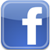找回密碼 密碼 註冊

# [教學] Matplotlib中的fill_between 用法發表於 2021-1-7 11:17:50 | 顯示全部樓層 |閱讀模式Matplotlib中的fill_between()函式總結  最近在處理資料的時候，需要從資料集合中選出資料來作為訓練集，不同的篩選規則得到的資料塊的分佈一樣，所以就想檢視一下選取了那些資料塊，比如將選中的資料換一種顏色來表示，但是資料如果比較多的話，就不太能看的出來了，所以如果將選中的資料塊以柱狀的形式框出來就好了，這個時候我看到了fill_between()函式，但是網上關於這個函式的部落格都寫的不太明白，所以我自己研究了一下，將其總結如下：1. 生成測試資料import numpy as np import matplotlib.pyplot as plt x = np.array([i for i in range(30)]) y = np.random.rand(30) plt.plot(x, y) plt.show()複製代碼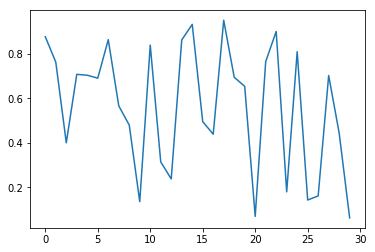2. 曲線覆蓋  我隨機生成了上圖所示的資料，現在我想將曲線下面的部分全部覆蓋為綠色，這個時候只需要呼叫函式fill_between()就可以實現：plt.plot(x, y)   # 先將圖畫出來 plt.fill_between(x, 0, y, facecolor='green', alpha=0.3) plt.show()複製代碼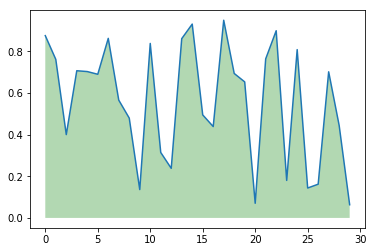可以看到，我用了這個函式的幾個引數： x：第一個引數表示覆蓋的區域，我直接複製為x，表示整個x都覆蓋 0：表示覆蓋的下限 y：表示覆蓋的上限是y這個曲線 facecolor：覆蓋區域的顏色 alpha：覆蓋區域的透明度[0,1],其值越大，表示越不透明3. 部分割槽域覆蓋 plt.plot(x, y)   # 先將圖畫出來 plt.fill_between(x[2:15], 0.2, 0.6, facecolor='green', alpha=0.3) plt.show()複製代碼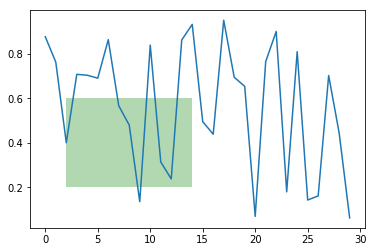我將x改為x[2:15],y改成了0.6，這樣它就在x軸的214，y軸的0.20.6畫出了這樣一個矩形4. 兩曲線之間的部分填充 y1 = np.random.rand(30)  # 生成第一條曲線 y2 = y1 + 0.3            # 生成第二條曲線 plt.plot(x, y1, 'b') plt.plot(x, y2, 'r') plt.fill_between(x, y1, y2, facecolor='green', alpha=0.3) plt.show()複製代碼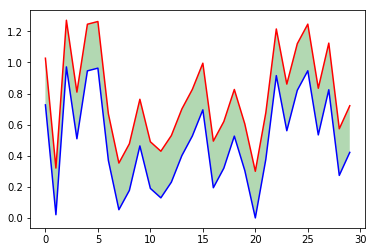把下限換成y1,上限換成y2,在整個x軸上進行填充，這樣就可以實現將兩條曲線之間的部分進行填充。5. 我想實現的資料高亮功能 x = np.array([i for i in range(30)]) y = np.random.rand(30) # 設定想要高亮資料的位置 position = [[1, 6],             [10, 12],             [20, 23],             [26, 28]] # 畫圖 plt.plot(x, y, 'r') for i in position:     plt.fill_between(x[ i : i ], 0, 1, facecolor='green', alpha=0.3) plt.show()複製代碼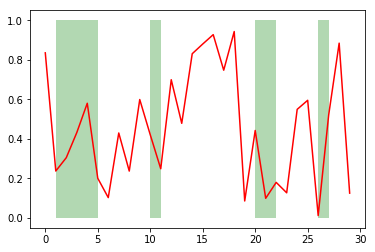只需要迴圈填充你想要填充的部分，設定填充的上下限，就可以實現這個功能了！ 文章出處

Matplotlib fill_between pythonMatplotlib fill_between pythonMatplotlib fill_between python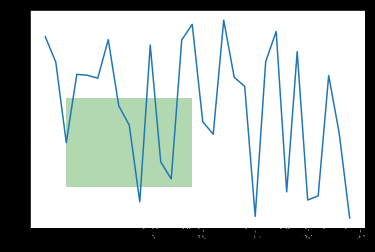Matplotlib fill_between python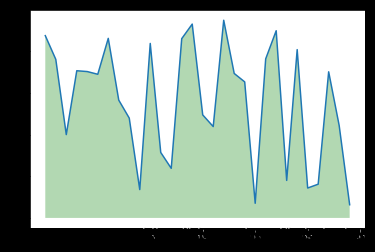Matplotlib fill_between python本版積分規則 回帖後跳轉到最後一頁
﻿

GMT+8, 2021-1-22 04:04 , Processed in 0.065750 second(s), 24 queries .